Courses

# Torsion of Circular Shafts Notes | EduRev

## Civil Engineering (CE) : Torsion of Circular Shafts Notes | EduRev

The document Torsion of Circular Shafts Notes | EduRev is a part of the Civil Engineering (CE) Course Solid Mechanics.
All you need of Civil Engineering (CE) at this link: Civil Engineering (CE)

Uniform Torsion

1. Torsion of Shaft and Combined Stresses
Torsion means twisting a structural Member when it is loaded by a couple that Produces rotation about the longitudinal axis.
If  τ be the intensity of shear stress, on any layer at a distance r from the centre of shaft, then
τ/ r = T / J = Gθ / l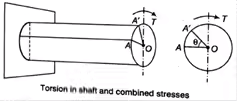2. Sign Convention
(i) Sign convention of torque can be explained by right hand thumb rule.
(ii) A positive torque is that in which there is tightening effect of nut on the bolt. From either side of the cross-section. If torque is applied in the direction of right hand fingers than right hand thumbs direction represents movement of the nut.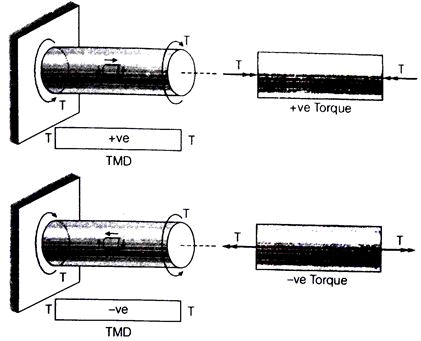TMD = Torsion moment diagram
T = Torque
Total angle of twist :
θ = Tl / GJ
Where, T = Torque,
J = Polar moment of inertia
G = Modulus of rigidity,
θ = Angle of twist
L = Length of shaft,
GJ = Torsional rigidity
GJ / l → Torsional stiffness;
l / GJ → Torsional flexibility
EA / l → Axial stiffness
l / EA → Axial flexibility
3. Moment of Inertia About polar Axis
(i) For solid circular shaft: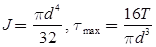(ii) For hollow circular shaft: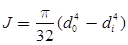4. Power Transmitted in the Shaft
(i) Power transmitted by shaft:
P = (2πNT / 60000)kW
Where, N = Rotation per minute.
5. Compound Shaft
An improved type of compound coupling for connecting in series and parallel are given below
(i) Series connection: Series connection of compound shaft as shown in figure. Due to series connection the torque on shaft 1 will be equal to shaft 2 and the total angular deformation will be equal to the sum of deformation of 1st shaft and 2nd shaft.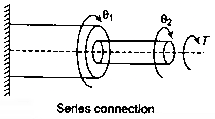θ = θ1 + θ2
T = T+ T2
Therefore,
θ = TL1 / G1J1 + TL2 / G2J2
Where,
θ= Angular deformation of 1st shaft
θ2 = Angular deformation of 2nd shaft
(ii) Parallel connection: Parallel connection of compound shaft as shown in figure. Due to parallel connection of compound shaft the total torque will be equal to the sum of torque of shaft 1 and torque of shaft 2 and the deflection will be same in both the shafts.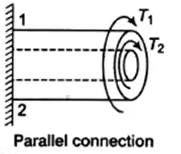θ= θ2
T = T1 + T2
Therefore,
T1L / G1J1 = T2L / G2J2
6. Strain energy (U) stored in shaft due to torsion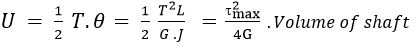(i) G = Shear modulus
(ii) T = Torque
(iii) J = Moment of inertia about polar axis
7. Effect of Pure Bending on Shaft
The effect of pure bending on shaft can be defined by the relation for the shaft,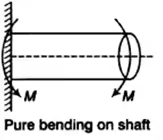σ = 32M / πD3
Where, σ = Principal stress
D = Diameter of shaft
M = Bending moment
8. Effect of Pure Torsion on Shaft
It can be calculated by the formula, which are given below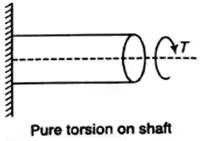τmax = 16T/πD3
Where, τ = Torsion
D = Diameter of shaft
9. Combined effect of bending and torsion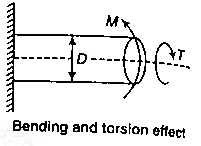(i) Principal stress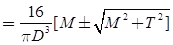(ii) Maximum shear stress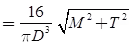(iii) Equivalent bending moment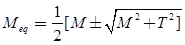(iv) Equivalent torque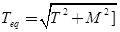10. Shear Stress Distribution
(i) Solid Circulation Section: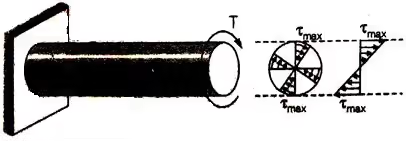(ii) Hollow Circulation Section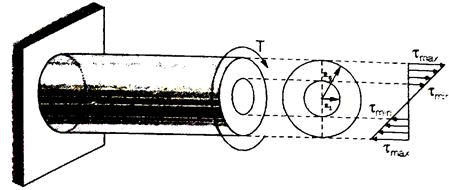(iii) Composite Circular Section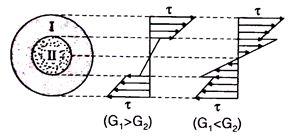(iv) Thin Tubular section: In view of small thickness-shear stress is assumed to be uniform.
Offer running on EduRev: Apply code STAYHOME200 to get INR 200 off on our premium plan EduRev Infinity!

## Solid Mechanics

30 videos|27 docs|29 tests

,

,

,

,

,

,

,

,

,

,

,

,

,

,

,

,

,

,

,

,

,

;